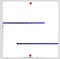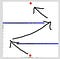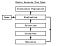# The Genetic Algorithm –Explained With “Intelligent” Dots

Artificial Intelligence is going to take over the world. Either through supreme AI overloads (like the ones commonly depicted in sci-fi dystopian movies) or simply by automating nearly the entire workforce, we all know it’s bound to happen.

This justifies the importance of understanding this technology and how it can be created. This article will go over one of the key methods behind machine learning, The Genetic Algorithm.

For a simple example, let’s say we want to make an artificial intelligence that can move a dot between two points as efficiently as possible, how would we do that??

Hmm… here’s an idea! What if we made a program that mimics the principles of biological evolution?

Genetic algorithms are one of the many various approaches used in machine learning (ML). They can be used to derive solutions to machine learning problems and optimize the produced models (solutions).

The genetic algorithm is one of the most fundamental algorithms used in machine learning. It mimics biological evolution in order to develop advanced solutions to data analysis problems. It’s based on the idea that just like in biology machine learning models should “evolve” (or train/develop) in order to become better at solving their problems.

What separates the genetic algorithm from many of the other ML methods is that with many of them such as gradient descent which is used for image classification models when you look into how they work they’re really just fancy math equations.

With genetic algorithms, however, we leverage random chance and the Darwinian principle of survival of the fittest to develop accurate models without the use of complicated math. In some ways, this feels a lot more like biological learning, rather than just math (although it’s still miles away from being anywhere close to true general intelligence).

To explain the methodology behind genetic algorithms its best to look at an example, so let’s explore the problem mentioned before of making a dot move between two points.

Let’s say we have a map that looks like the one below, and we want to make a dot go from the red dot at the bottom of the frame to the red dot at the top without touching the walls or the blue obstacles.How can we make a dot move between the two red dots?

We might be able to use our eyes and brains to easily see the shortest route. However, a computer can’t just “see” the answer as we can. It has to figure it out somehow.We may be able to “see” that this is the route the dot should take, but the computer can’t

When applying the genetic algorithm the general process follows the flow chart below.If this doesn’t make sense right now, it’s ok it should make more sense after the example

So, if the genetic algorithm were to be applied to our problem, the pseudo code would go something like this.

1. Instantiate a population of 1000 dots
2. Generate random steps for each individual dot
3. Have each dot take random steps until they hit a wall and die
4. Calculate the fitness
5. Use the parents to generate the next generation of 1000 dots
6. Repeat until you’re satisfied.

It’s understandable if this seems a bit confusing so let’s go over it further with the related code.

# Instantiate a population of 1000 dots

All this does is it creates our population of dots which will be used for evolution. For our algorithm, we’re using a population of 1000, although theoretically this could be changed to any number. It might be your first instinct to make the population as large as possible. This would allow for more variation in each generation which can possibly allow our model to discover the best path quicker. However, increasing the population size also increases the time it takes to run each generation. This can possibly have the opposite effect and slow down the training process. Like with many things in life, the solution to this is finding the right balance. This means experimenting with different values and finding a good balance between population size and speed.

We can create our population with the following code. This method takes in a variable representing the desired population size and then creates a population of that given size. This population is stored inside an array to make it easy to manipulate.

# Generate random steps for each individual dot

A step is simply a move taken by the dot in the plane. In this program, the steps are determined by changes in velocity and direction. For example, for the duration of one step the dot could be moving up, but then in the next step, it could be moving in the opposite direction, down.

If we continue applying the analogy of genetic evolution to this program, the specific steps taken by each dot can be thought of like each one’s individual DNA. Therefore, because each dot has a different record of the steps it took, each dot has different DNA. If you recall from your biology classes, this genetic variation allows for evolution through the increased expression of good traits and elimination of bad traits between generations.

Creating the random steps taken by each dot in the population looks like this in code. The directions array holds the different steps for each dot. It can be thought of as a thing which contains each dots DNA.

# Have each dot take random steps until they hit a wall and die

By having each dot take random steps we are going to end up with a bunch of random possible paths the dots can take. On the first round, unfortunately, all of these will be awful and all the dots will most likely end up dying by hitting a wall. However, that’s okay! This is because the model will soon improve.

The theory behind this is that out of the 1000 different paths (1 for each dot in the population) we can select the best paths and use them as the base paths for the next generation. The next generation (already having good paths as starting points) will be able to further refine it and find a slightly better one just through chance.

Moving the dots during each generation looks like this in code.

# Calculate the fitness

Biology is said to be survival of the fittest, this is no different. In the process of evolution, fitness is a measurement of how good an organism is at surviving and reproducing in its environment. A higher fitness results in a higher probability of the organism passing on its genes to the next generation. This is known as natural selection and is the driving force behind evolution.

In our scenario, we are also going to use fitness to determine which dots get to pass their genes on to the next generation. If only the dots with the best genes pass their genes on, it means that each generation will progressively improve.

For our purposes, fitness will be determined by how close a dot gets to the goal and by how many steps it can do it in. The closer a dot can get, the higher the fitness. The fewer steps it takes to get there, the higher the fitness.

The fitness of each dot in the generation is calculated with the following code.

# Use the parents to generate the next generation of 1000 dots

In the process of evolution, populations pass on their best genes to the next generation. Once again, this is no different.

The following code allows us to create a new generation from the previous one by mimicking the process of “natural selection”.

After calculating the fitness of each dot in the population, we can then use each one’s fitness to determine their individual probability of passing on their genes to the next generation. (The higher the fitness, the higher the probability their genes will get passed on.)

In the new generation, we have a new population of 1000 dots. Each one of the dots will receive their DNA (steps) directly from a parent in the previous generation. Recall that we’ve already calculated the probability that these parent dots have of passing on their genes. Now, using these probabilities, we’re able to assign the children to parents and give them their base DNA.

The number of children a parent dot passes its genes to should correspond with the probability it had of passing its gene’s on. Let’s say a dot had a probability to pass on its genes of around 40%, this means approximately 40 of the 100 new dots would be children of this parent.

Selection based on these probabilities is performed by the following code.

However, just doing this wouldn’t allow for any improvement over the past generation, because each dot would be identical to their parent!

To solve this we “mutate” some of the genes in each dot (we change random steps in each DNA sequence). This allows dots of this generation to take new paths, some of which are hopefully better and can be used as a base for the next generation, providing for continuous improvement.

The mutation is performed by the following code for each dot’s DNA.

# Repeat until you’re satisfied

Repeating the above process will result in the best path continuously being refined until the model finds the shortest path it can and no more improvements or reductions to the step count can be made.

The following code is responsible for cycling through the process and training the AI.

Voilà! We’ve just finished making an AI that is able to find a path between two points by “learning”.

This example, while requiring many parts to solve a seemingly easy task, is actually a very simplistic implementation of a genetic algorithm. Despite this, for our applications, this algorithm is efficient enough and actually quite satisfactory.

More complicated versions of genetic algorithms also exist. For example, there are some implementations which elect to splice information between the top two models in order to derive better children from them and create more genetic variation. This is in contrast to how we just created the children directly from one parent and then used mutations to induce more variation.

Even though these more complicated versions exist, it doesn’t mean you should always use them. With machine learning, it’s very important to remember that each problem is unique and that the best solution will often be one specifically tailored to the problem.

This is why genetic algorithms that splice information between the top two models can be useful for some applications, but completely unnecessary with ours.

Key Takeaway

The genetic algorithm is one of many machine learning methods. It’s often not the most efficient way to generate a machine learning model but it is nonetheless effective, and for problems where you might not be aware of a better method, it is often easy to apply this method which will likely provide good results.

On an android phone? Download this app from my GitHub to test out the dots yourself! If you’re on a computer, you can also find the code for this project here.

Before you go